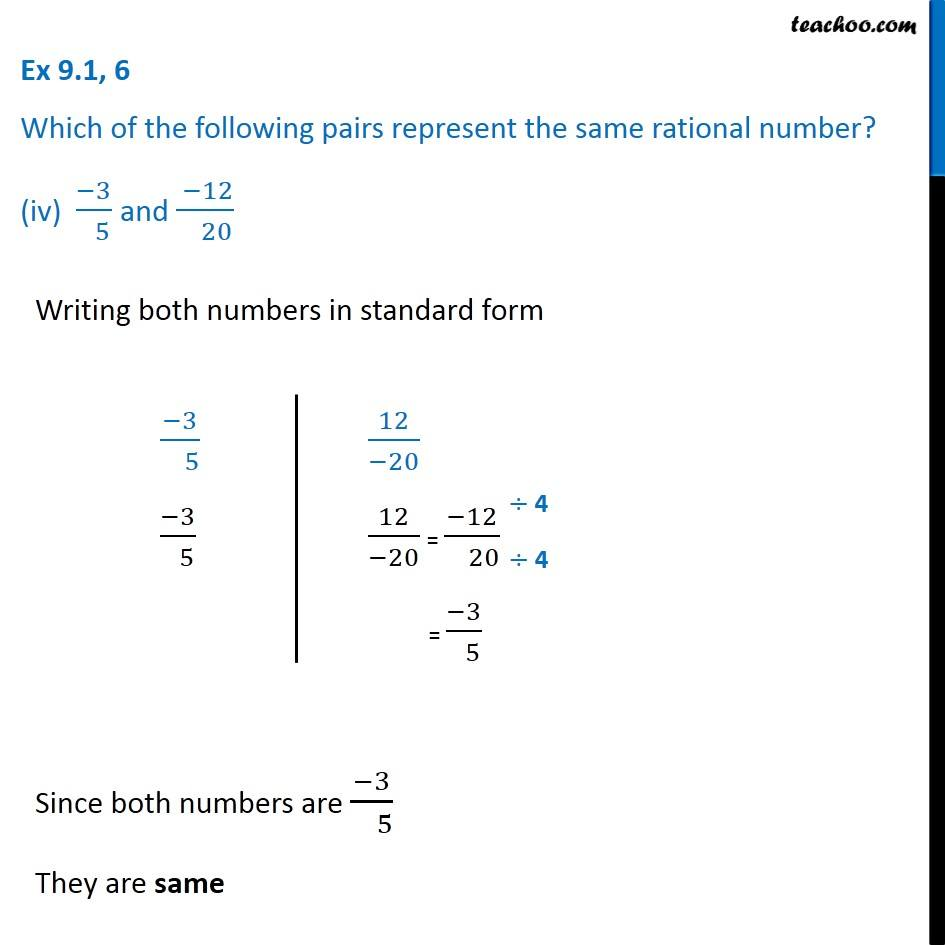Ex 9.1

Chapter 9 Class 7 Rational Numbers
Serial order wiseIntroducing your new favourite teacher - Teachoo Black, at only ₹83 per month

### Transcript

Ex 9.1, 6 Which of the following pairs represent the same rational number? (iv) (−3)/( 5) and ( −12)/( 20) Writing both numbers in standard form (−3)/( 5) (−3)/( 5) 12/(−20) 12/(−20) = (−12)/( 20) = (−3)/( 5) Since both numbers are (−3)/( 5) They are same